# Electromagnet

﻿
Electromagnet

An electromagnet is a type of magnet in which the magnetic field is produced by the flow of electric current. The magnetic field disappears when the current is turned off. Electromagnets are widely used as components of other electrical devices, such as motors, generators, relays, loudspeakers, hard disks, MRI machines, scientific instruments, and magnetic separation equipment, as well as being employed as industrial lifting electromagnets for picking up and moving heavy iron objects like scrap iron.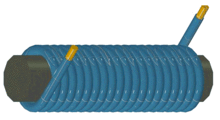A simple electromagnet consisting of a coil of insulated wire wrapped around an iron core. The strength of magnetic field generated is proportional to the amount of current.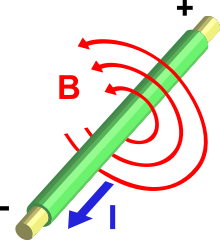Current (I) through a wire produces a magnetic field (B). The field is oriented according to the right-hand rule.

An electric current flowing in a wire creates a magnetic field around the wire (see drawing below). To concentrate the magnetic field, in an electromagnet the wire is wound into a coil, with many turns of wire lying side by side. The magnetic field of all the turns of wire passes through the center of the coil, creating a strong magnetic field there. A coil forming the shape of a straight tube (a helix) is called a solenoid; a solenoid that is bent into a donut shape so that the ends meet is called a toroid. Much stronger magnetic fields can be produced if a "core" of ferromagnetic material, such as soft iron, is placed inside the coil. The ferromagnetic core increases the magnetic field to thousands of times the strength of the field of the coil alone, due to the high magnetic permeability μ of the ferromagnetic material. This is called a ferromagnetic-core or iron-core electromagnet.Magnetic field produced by a solenoid (coil of wire). This drawing shows a cross section through the center of the coil. The crosses are wires in which current is moving into the page; the dots are wires in which current is moving up out of the page.

The direction of the magnetic field through a coil of wire can be found from a form of the right-hand rule. If the fingers of the right hand are curled around the coil in the direction of current flow (conventional current, flow of positive charge) through the windings, the thumb points in the direction of the field inside the coil. The side of the magnet that the field lines emerge from is defined to be the north pole.

The main advantage of an electromagnet over a permanent magnet is that the magnetic field can be rapidly manipulated over a wide range by controlling the amount of electric current. However, a continuous supply of electrical energy is required to maintain the field.

## How the iron core works

The material of the core of the magnet (usually iron) is composed of small regions called magnetic domains that act like tiny magnets (see ferromagnetism). Before the current in the electromagnet is turned on, the domains in the iron core point in random directions, so their tiny magnetic fields cancel each other out, and the iron has no large scale magnetic field. When a current is passed through the wire wrapped around the iron, its magnetic field penetrates the iron, and causes the domains to turn, aligning parallel to the magnetic field, so their tiny magnetic fields add to the wire's field, creating a large magnetic field that extends into the space around the magnet. The larger the current passed through the wire coil, the more the domains align, and the stronger the magnetic field is. Finally all the domains are lined up, and further increases in current only cause slight increases in the magnetic field: this phenomenon is called saturation.

When the current in the coil is turned off, most of the domains lose alignment and return to a random state and the field disappears. However some of the alignment persists, because the domains have difficulty turning their direction of magnetization, leaving the core a weak permanent magnet. This phenomenon is called hysteresis and the remaining magnetic field is called remanent magnetism. The residual magnetization of the core can be removed by degaussing.

## History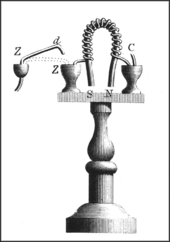Sturgeon's electromagnet, 1824

Danish scientist Hans Christian Ørsted discovered in 1820 that electric currents create magnetic fields. British scientist William Sturgeon invented the electromagnet in 1824. His first electromagnet was a horseshoe-shaped piece of iron that was wrapped with about 18 turns of bare copper wire (insulated wire didn't exist yet). The iron was varnished to insulate it from the windings. When a current was passed through the coil, the iron became magnetized and attracted other pieces of iron; when the current was stopped, it lost magnetization. Sturgeon displayed its power by showing that although it only weighed seven ounces (roughly 200 grams), it could lift nine pounds (roughly 4 kilos) when the current of a single-cell battery was applied. However, Sturgeon's magnets were weak because the uninsulated wire he used could only be wrapped in a single spaced out layer around the core, limiting the number of turns. Beginning in 1827, US scientist Joseph Henry systematically improved and popularized the electromagnet. By using wire insulated by silk thread he was able to wind multiple layers of wire on cores, creating powerful magnets with thousands of turns of wire, including one that could support 2,063 lb (936 kg). The first major use for electromagnets was in telegraph sounders.

The magnetic domain theory of how ferromagnetic cores work was first proposed in 1906 by French physicist Pierre-Ernest Weiss, and the detailed modern quantum mechanical theory of ferromagnetism was worked out in the 1920s by Werner Heisenberg, Lev Landau, Felix Bloch and others.

## Uses of electromagnets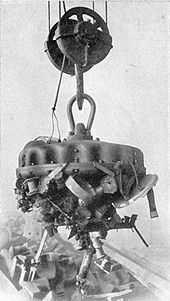Industrial electromagnet lifting scrap iron, 1914

Electromagnets are very widely used in electric and electromechanical devices, including:

## Analysis of ferromagnetic electromagnets

For definitions of the variables below, see box at end of article.

The magnetic field of electromagnets in the general case is given by Ampere's Law:$\int \mathbf{J}\cdot d\mathbf{A} = \oint \mathbf{H}\cdot d\mathbf{l}$

which says that the integral of the magnetizing field H around any closed loop of the field is equal to the sum of the current flowing through the loop. Another equation used, that gives the magnetic field due to each small segment of current, is the Biot-Savart law. Computing the magnetic field and force exerted by ferromagnetic materials is difficult for two reasons. First, because the strength of the field varies from point to point in a complicated way, particularly outside the core and in air gaps, where fringing fields and leakage flux must be considered. Second, because the magnetic field B and force are nonlinear functions of the current, depending on the nonlinear relation between B and H for the particular core material used. For precise calculations, computer programs that can produce a model of the magnetic field using the finite element method are employed.

### Magnetic circuit – the constant B field approximation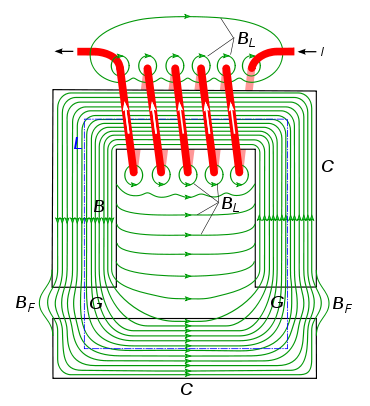Magnetic field (green) of a typical electromagnet, with the iron core C forming a closed loop with two air gaps G in it. Most of the magnetic field B is concentrated in the core. However some of the field lines BL, called the "leakage flux", do not follow the full core circuit and so do not contribute to the force exerted by the electromagnet. In the gaps G the field lines spread out beyond the boundaries of the core in "fringing fields" BF. This increases the "resistance" (reluctance) of the magnetic circuit, decreasing the total magnetic flux in the core. Both the leakage flux and the fringing fields get larger as the gaps are increased, reducing the force exerted by the magnet. Line L shows the average length of the magnetic circuit, used in equation (1) below. It is the sum of the length Lcore in the iron core and the length Lgap in the air gaps

In many practical applications of electromagnets, such as motors, generators, transformers, lifting magnets, and loudspeakers, the iron core is in the form of a loop or magnetic circuit, possibly broken by a few narrow air gaps. This is because iron presents much less "resistance" (reluctance) to the magnetic field than air, so a stronger field can be obtained if most of the magnetic field's path is within the core.

Since most of the magnetic field is confined within the outlines of the core loop, this allows a simplification of the mathematical analysis. See the drawing at right. A common simplifying assumption satisfied by many electromagnets, which will be used in this section, is that the magnetic field strength B is constant around the magnetic circuit and zero outside it. Most of the magnetic field will be concentrated in the core material (C). Within the core the magnetic field (B) will be approximately uniform across any cross section, so if in addition the core has roughly constant area throughout its length, the field in the core will be constant. This just leaves the air gaps (G), if any, between core sections. In the gaps the magnetic field lines are no longer confined by the core, so they 'bulge' out beyond the outlines of the core before curving back to enter the next piece of core material, reducing the field strength in the gap. The bulges (BF) are called fringing fields. However, as long as the length of the gap is smaller than the cross section dimensions of the core, the field in the gap will be approximately the same as in the core. In addition, some of the magnetic field lines (BL) will take 'short cuts' and not pass through the entire core circuit, and thus will not contribute to the force exerted by the magnet. This also includes field lines that encircle the wire windings but do not enter the core. This is called leakage flux. Therefore the equations in this section are valid for electromagnets for which:

1. the magnetic circuit is a single loop of core material, possibly broken by a few air gaps
2. the core has roughly the same cross sectional area throughout its length.
3. any air gaps between sections of core material are not large compared with the cross sectional dimensions of the core.
4. there is negligible leakage flux

The main nonlinear feature of ferromagnetic materials is that the B field saturates at a certain value, which is around 1.6 teslas (T) for most high permeability core steels. The B field increases quickly with increasing current up to that value, but above that value the field levels off and becomes almost constant, regardless of how much current is sent through the windings. So the strength of the magnetic field possible from an iron core electromagnet is limited to around 1.6 to 2 T.[citation needed]

### Magnetic field created by a current

The magnetic field created by an electromagnet is proportional to both the number of turns in the winding, N, and the current in the wire, I, hence this product, NI, in ampere-turns, is given the name magnetomotive force. For an electromagnet with a single magnetic circuit, of which length Lcore is in the core material and length Lgap is in air gaps, Ampere's Law reduces to:$NI = H_{\mathrm{core}} L_{\mathrm{core}} + H_{\mathrm{gap}} L_{\mathrm{gap}}\,$$NI = B \left(\frac{L_{\mathrm{core}}}{\mu} + \frac{L_{\mathrm{gap}}}{\mu_0} \right) \qquad \qquad \qquad \qquad (1) \,$
where$\mu = B/H\,$$\mu_0 = 4 \pi (10^{-7}) \ \mathrm{N} \cdot \mathrm{A}^{-2}$ is the permeability of free space (or air); note that A in this definition is amperes.

This is a nonlinear equation, because the permeability of the core, μ, varies with the magnetic field B. For an exact solution, the value of μ at the B value used must be obtained from the core material hysteresis curve. If B is unknown, the equation must be solved by numerical methods. However, if the magnetomotive force is well above saturation, so the core material is in saturation, the magnetic field will be approximately the saturation value Bsat for the material, and won't vary much with changes in NI. For a closed magnetic circuit (no air gap) most core materials saturate at a magnetomotive force of roughly 800 ampere-turns per meter of flux path.

For most core materials,$\mu_r = \mu / \mu_0 \approx 2000 - 6000\,$. So in equation (1) above, the second term dominates. Therefore, in magnetic circuits with an air gap, the strength of the magnetic field B depends strongly on the length of the air gap, and the length of the flux path in the core doesn't matter much.

### Force exerted by magnetic field

The force exerted by an electromagnet on a section of core material is:$F = \frac{B^2 A}{2 \mu_0} \qquad \qquad \qquad \qquad \qquad \qquad (2) \,$

The 1.6 T limit on the field mentioned above sets a limit on the maximum force per unit core area, or pressure, an iron-core electromagnet can exert; roughly:$\frac{F}{A} = \frac {B_{sat}^2}{2 \mu_0} \approx 1000\ \mathrm{kPa} = 10^6 \mathrm{N/m^2} = 145\ \mathrm{lbf} \cdot \mathrm{in}^{-2}\,$

In more intuitive units it's useful to remember that at 1T the magnetic pressure is approximately 4 atmospheres, or kg/cm2.

Given a core geometry, the B field needed for a given force can be calculated from (2); if it comes out to much more than 1.6 T, a larger core must be used.

### Closed magnetic circuit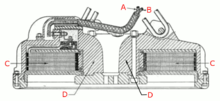Cross section of lifting electromagnet like that in above photo, showing cylindrical construction. The windings (C) are flat copper strips to withstand the Lorentz force of the magnetic field. The core is formed by the thick iron housing (D) that wraps around the windings.

For a closed magnetic circuit (no air gap), such as would be found in an electromagnet lifting a piece of iron bridged across its poles, equation (1) becomes:$B = \frac{NI\mu}{L} \qquad \qquad \qquad \qquad \qquad \qquad (3) \,$

Substituting into (2), the force is:$F = \frac{\mu^2 N^2 I^2 A}{2\mu_0 L^2} \qquad \qquad \qquad \qquad \qquad (4) \,$

It can be seen that to maximize the force, a core with a short flux path L and a wide cross sectional area A is preferred. To achieve this, in applications like lifting magnets (see photo above) and loudspeakers a flat cylindrical design is often used. The winding is wrapped around a short wide cylindrical core that forms one pole, and a thick metal housing that wraps around the outside of the windings forms the other part of the magnetic circuit, bringing the magnetic field to the front to form the other pole.

### Force between electromagnets

The above methods are inapplicable when most of the magnetic field path is outside the core. For electromagnets (or permanent magnets) with well defined 'poles' where the field lines emerge from the core, the force between two electromagnets can be found using the 'Gilbert model' which assumes the magnetic field is produced by fictitious 'magnetic charges' on the surface of the poles, with pole strength m and units of Ampere-turn meter. Magnetic pole strength of electromagnets can be found from:$m = \frac{NIA}{L}$

The force between two poles is:$F = \frac{\mu_0 m_1 m_2}{4\pi r^2}$

This model doesn't give the correct magnetic field inside the core, and thus gives incorrect results if the pole of one magnet gets too close to another magnet.

## Side effects in large electromagnets

There are several side effects which become important in large electromagnets and must be provided for in their design:

### Ohmic heating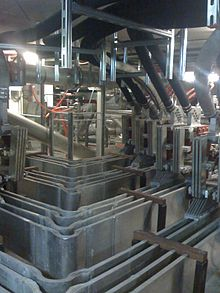Large aluminum busbars carrying current into the electromagnets at the LNCMI (Laboratoire National des Champs Magnétiques Intenses) high field laboratory.

The only power consumed in a DC electromagnet is due to the resistance of the windings, and is dissipated as heat. Some large electromagnets require cooling water circulating through pipes in the windings to carry off the waste heat.

Since the magnetic field is proportional to the product NI, the number of turns in the windings N and the current I can be chosen to minimize heat losses, as long as their product is constant. Since the power dissipation, P = I2R, increases with the square of the current but only increases approximately linearly with the number of windings, the power lost in the windings can be minimized by reducing I and increasing the number of turns N proportionally. For example halving I and doubling N halves the power loss. This is one reason most electromagnets have windings with many turns of wire.

However, the limit to increasing N is that the larger number of windings takes up more room between the magnet's core pieces. If the area available for the windings is filled up, more turns require going to a smaller diameter of wire, which has higher resistance, which cancels the advantage of using more turns. So in large magnets there is a minimum amount of heat loss that can't be reduced. This increases with the square of the magnetic flux B2.

### Inductive voltage spikes

An electromagnet is a large inductor, and resists changes in the current through its windings. Any sudden changes in the winding current cause large voltage spikes across the windings. This is because when the current through the magnet is increased, such as when it is turned on, energy from the circuit must be stored in the magnetic field. When it is turned off the energy in the field is returned to the circuit.

If an ordinary switch is used to control the winding current, this can cause sparks at the terminals of the switch. This doesn't occur when the magnet is switched on, because the voltage is limited to the power supply voltage. But when it is switched off, the energy in the magnetic field is suddenly returned to the circuit, causing a large voltage spike and an arc across the switch contacts, which can damage them. With small electromagnets a capacitor is often used across the contacts, which reduces arcing by temporarily storing the current. More often a diode is used to prevent voltage spikes by providing a path for the current to recirculate through the winding until the energy is dissipated as heat. The diode is connected across the winding, oriented so it is reverse-biased during steady state operation and doesn't conduct. When the supply voltage is removed, the voltage spike forward-biases the diode and the reactive current continues to flow through the winding, through the diode and back into the winding. A diode used in this way is often called a flyback diode.

Large electromagnets are usually powered by variable current electronic power supplies, controlled by a microprocessor, which prevent voltage spikes by accomplishing current changes in gentle ramps. It may take several minutes to energize or deenergize a large magnet.

### Lorentz forces

In powerful electromagnets, the magnetic field exerts a force on each turn of the windings, due to the Lorentz force$q\mathbf{v}\times\mathbf{B}\,$ acting on the moving charges within the wire. The Lorentz force is perpendicular to both the axis of the wire and the magnetic field. It can be visualized as a pressure between the magnetic field lines, pushing them apart. It has two effects on an electromagnet's windings:

• The field lines within the axis of the coil exert a radial force on each turn of the windings, tending to push them outward in all directions. This causes a tensile stress in the wire.
• The leakage field lines between each turn of the coil exert a repulsive force between adjacent turns, tending to push them apart.

The Lorentz forces increase with B2. In large electromagnets the windings must be firmly clamped in place, to prevent motion on power-up and power-down from causing metal fatigue in the windings. In the Bitter design, below, used in very high field research magnets, the windings are constructed as flat disks to resist the radial forces, and clamped in an axial direction to resist the axial ones.

### Core losses

In alternating current (AC) electromagnets, used in transformers, inductors, and AC motors and generators, the magnetic field is constantly changing. This causes energy losses in their magnetic cores that are dissipated as heat in the core. The losses stem from two processes:

• Eddy currents: From Faraday's law of induction, the changing magnetic field induces circulating electric currents inside nearby conductors, called eddy currents. The energy in these currents is dissipated as heat in the electrical resistance of the conductor, so they are a cause of energy loss. Since the magnet's iron core is conductive, and most of the magnetic field is concentrated there, eddy currents in the core are the major problem. Eddy currents are closed loops of current that flow in planes perpendicular to the magnetic field. The energy dissipated is proportional to the area enclosed by the loop. To prevent them, the cores of AC electromagnets are made of stacks of thin steel sheets, or laminations, oriented parallel to the magnetic field, with an insulating coating on the surface. The insulation layers prevent eddy current from flowing between the sheets. Any remaining eddy currents must flow within the cross section of each individual lamination, which reduces losses greatly. Another alternative is to use a ferrite core, which is a nonconductor.
• Hysteresis losses: Reversing the direction of magnetization of the magnetic domains in the core material each cycle causes energy loss, because of the coercivity of the material. These losses are called hysteresis. The energy lost per cycle is proportional to the area of the hysteresis loop in the BH graph. To minimize this loss, magnetic cores used in transformers and other AC electromagnets are made of "soft" low coercivity materials, such as silicon steel or soft ferrite.

The energy loss per cycle of the AC current is constant for each of these processes, so the power loss increases linearly with frequency.

## High field electromagnets

### Superconducting electromagnets

When a magnetic field higher than the ferromagnetic limit of 1.6 T is needed, superconducting electromagnets can be used. Instead of using ferromagnetic materials, these use superconducting windings cooled with liquid helium, which conduct current without electrical resistance. These allow enormous currents to flow, which generate intense magnetic fields. Superconducting magnets are limited by the field strength at which the winding material ceases to be superconducting. Current designs are limited to 10–20 T, with the current (2009) record of 33.8 T. The necessary refrigeration equipment and cryostat make them much more expensive than ordinary electromagnets. However, in high power applications this can be offset by lower operating costs, since after startup no power is required for the windings, since no energy is lost to ohmic heating. They are used in particle accelerators, MRI machines, and research.

### Bitter electromagnets

Both iron-core and superconducting electromagnets have limits to the field they can produce. Therefore the most powerful man-made magnetic fields have been generated by air-core nonsuperconducting electromagnets of a design invented by Francis Bitter in 1933, called Bitter electromagnets. Instead of wire windings, a Bitter magnet consists of a solenoid made of a stack of conducting disks, arranged so that the current moves in a helical path through them. This design has the mechanical strength to withstand the extreme Lorentz forces of the field, which increase with B2. The disks are pierced with holes through which cooling water passes to carry away the heat caused by the high current. The strongest continuous field achieved with a resistive magnet is currently (2008) 35 T, produced by a Bitter electromagnet. The strongest continuous magnetic field, 45 T, was achieved with a hybrid device consisting of a Bitter magnet inside a superconducting magnet.

### Exploding electromagnets

The factor limiting the strength of electromagnets is the inability to dissipate the enormous waste heat, so more powerful fields, up to 90 T, have been obtained from resistive magnets by sending brief pulses of current through them. The most powerful manmade magnetic fields have been created by using explosives to compress the magnetic field inside an electromagnet as it is pulsed. The implosion compresses the magnetic field to values of around 1000 T for a few microseconds. While this method may seem very destructive there are methods to control the blast so that neither the experiment or the magnetic structure are harmed, by redirecting the brunt of the force radially outwards. These devices are known as destructive pulsed electromagnets. They are used in physics and materials science research to study the properties of materials at high magnetic fields.

## Definition of terms$A\,$ square meter cross sectional area of core$B\,$ tesla Magnetic field (Magnetic flux density)$F\,$ newton Force exerted by magnetic field$H\,$ ampere per meter Magnetizing field$I\,$ ampere Current in the winding wire$L\,$ meter Total length of the magnetic field path$L_{\mathrm{core}}+L_{\mathrm{gap}}\,$$L_{\mathrm{core}}\,$ meter Length of the magnetic field path in the core material$L_{\mathrm{gap}}\,$ meter Length of the magnetic field path in air gaps$m_1, m_2\,$ ampere meter Pole strength of the electromagnet$\mu\,$ newton per square ampere Permeability of the electromagnet core material$\mu_0\,$ newton per square ampere Permeability of free space (or air) = 4π(10−7)$\mu_r\,$ - Relative permeability of the electromagnet core material$N\,$ - Number of turns of wire on the electromagnet$r\,$ meter Distance between the poles of two electromagnets

Wikimedia Foundation. 2010.

### Look at other dictionaries:

• electromagnet — ELECTROMAGNÉT, electromagneţi, s.m. Bucată de oţel care capătă proprietăţi magnetice când, printr un fir metalic care o înfăşoară, trece un curent electric. ♦ Corp feromagnetic magnetizat temporar. – Din germ. Elektromagnet. Trimis de RACAI,… …   Dicționar Român

• electromagnet — 1831; see ELECTRO (Cf. electro ) + MAGNET (Cf. magnet) …   Etymology dictionary

• electromagnet — ► NOUN ▪ a metal core made into a magnet by the passage of electric current through a surrounding coil …   English terms dictionary

• electromagnet — [ē lek΄trō mag′nit, ilek′trō mag΄nit; ē lek′trəmag΄nit, i lek′trəmag΄nit] n. a soft iron or steel core surrounded by a coil of wire, that temporarily becomes a magnet when an electric current flows through the wire …   English World dictionary

• electromagnet — /i lek troh mag nit/, n. a device consisting of an iron or steel core that is magnetized by electric current in a coil that surrounds it. [1815 25; ELECTRO + MAGNET] * * * Device consisting of a core of magnetic material such as iron, surrounded… …   Universalium

• electromagnet — UK [ɪˌlektrəʊˈmæɡnət] / US [ɪˌlektroʊˈmæɡnət] noun [countable] Word forms electromagnet : singular electromagnet plural electromagnets physics a powerful magnet that uses an electric current passed in a wire around it to produce its force …   English dictionary

• electromagnet — [[t]ɪle̱ktroʊmæ̱gnɪt[/t]] electromagnets N COUNT An electromagnet is a magnet that consists of a piece of iron or steel surrounded by a coil. The metal becomes magnetic when an electric current is passed through the coil …   English dictionary

• electromagnet — elektromagnetas statusas T sritis automatika atitikmenys: angl. electromagnet vok. Elektromagnet, m rus. электромагнит, m pranc. électro aimant, m …   Automatikos terminų žodynas

• electromagnet — elektromagnetas statusas T sritis fizika atitikmenys: angl. electromagnet vok. Elektromagnet, m rus. электромагнит, m pranc. électro aimant, m …   Fizikos terminų žodynas

• electromagnet — noun Date: 1831 a core of magnetic material (as iron) surrounded by a coil of wire through which an electric current is passed to magnetize the core …   New Collegiate Dictionary